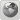Runiter Company
Would you like to react to this message? Create an account in a few clicks or log in to continue.

# 3D parametric equation with given points##3D parametric equation with given points

Hi,

In your 3d graphing calculator would it be possible to get 3D parametric equation to represent the graph of given x,y,z coordinates on 3D graph calculator program?

It seems that it is possible to plot x,y,z points and connect each point as a line to draw a parametric curve on this program, however what I'm looking for is something similar to the functionality on Microsoft Excel, which you plot x,y points and Excel draws a graph of parametric curve with given points and also gives you the equation of the curve.
But unfortunately Excel doesn't support this for 3D curves.

It would be great if your program can give the equation for the 3D graph drawn with points given.

Would this feature be something you can add?

Thanks,

greenfish

Posts : 1
Join date : 2013-04-07

##Re: 3D parametric equation with given points

Hi greenfish,
At the moment our software doesn't support that but this is an interesting suggestion and we will consider adding.
I will look at how excel does it and determine the feasibility of doing it in 3D.

Will let you know about whether or not it will be added to a future release.

_________________
Saeid Nourian, Ph.D.
Founder of Runiter Company
http://www.runiter.comruniter

Posts : 135
Join date : 2007-12-05
Age : 39
Location : Boston, MA, USAPermissions in this forum:
You cannot reply to topics in this forum